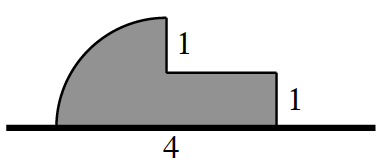### Home > APCALC > Chapter 5 > Lesson 5.1.1 > Problem5-11

5-11.

A horizontal flag, composed of a quarter-circle and a rectangle, is shown at right.

1. Imagine rotating the flag about its pole and describe the resulting three-dimensional figure. Draw a picture of this figure on your paper.

If the flag were rotated it would look like a mushroom.

2. Calculate the volume of the rotated flag.

Use geometry to find the volume of the resulting three-dimensional figure.

$V=\frac{1}{2}\text{ sphere + prism}$